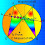## Tuesday, September 26, 2017

### Geometry Problem 1347: Triangle, Angle Bisector, Congruence, Circumcircle, Tangent, Equilateral Triangle

Geometry Problem. Post your solution in the comment box below.
Level: Mathematics Education, High School, Honors Geometry, College.

Details: Click on the figure below.1.∠BDC = ∠BCE = ∠BEC, so BDCE is concyclic.
=> ∠ADE = ∠EDC = ∠BDC = 60.

1.2.I thought it is obvious so I skip the explanation.
* ∠BDC = ∠BCE is because the triangle BDC & triangle BCA are similar under the power of circle at B (with C is the tangent point),
** ∠BCE = ∠BEC is because BE = CE so that triangle BCE is isos.

2.Let X be the foot of the perpendicular from E to AB and Y that of E to DC.

Triangles EXD and EYD are congruent ASA.

So EX = EY and hence triangles EXB and EYC are congruent right angle, hypotenuse, side.

It follows that < DBE = < DCE and hence BCED is concyclic and so < C = < BCE = < EBC = < BDC = < BEC

Therefore Tr. BCE is equilateral

Sumith Peiris
Moratuwa
Sri Lanka

1.To Sumith
1. Your proof never use the given data " BC tangent to the circumcircle of triangle ADC at C "
2. referring to line 4 of your solution "
It follows that < DBE = < DCE and hence BCED is concyclic and so < C = < BCE = < EBC = < BDC = < BEC "
Please explain how do you get < EBC=<BDC

Peter Tran

2.Peter

Because of the tangency,
<A = DAC = DCB and hence Tr.s ABC & CBD are similar and so
< BDC = C.

But < EBC = < ECB = C
Hence < EBC = < BDC

Hope this clarifies

Sumith

3.Once you prove BCED is concyclic, without additional construction we can prove BEC as equilateral triangle by using alternate-segment theorem.

Suppose say the m(BCA) = x => m(ADC) = 180-x => m(CDB) = x
Since BCED is concyclic, m(CEB) = m(CDB) = x and hence BCE is equilateral triangle.

4.Repeating my response to Peter's comments

1- I do, in fact,use the data on the tangency which gives < A = < BCD
2 - As a result of the 1- above Tr.s of ABC and CBD are similar. Hence < BDC = <BCE = < EBC.

Trust this clarifies

Sumith

5.Thanks for the clarification

Peter Tran

3.https://photos.app.goo.gl/UZHIJKvyxwGfqzUG3

Let ∠ (ADE)= ∠ (EDC)= u
Let X and Y are the projection of E over AD and DC
Triangle BEX congruent to CEY => ∠ (XBE)= ∠ (ECY) => quadrilateral BDEC is cyclic
Let DE cut circumcircle of triangle ADC at F .
F will be the midpoint of arc AFC and ∠ (FAC)= ∠ (FCA)= u
Since BC tangent to circle ADC => ∠ (AFC)= ∠ (ACB)= u
So AFC is a equilateral triangle and u= 60 degrees
So EBC is also a equilateral triangle

4.Let's call ang(BAC)=y, ang(ECB)=ang(BEC)=u, and ang(ADE)=ang(EDB)=x.
1. ang(CBD)=y because of BC tangent.
2. Notice in the intersection of CE and DB that 180°-u-y=180°+u-2x-y. Then we have u=x.
3. DCBE is a cyclic quadrilateral because diagonal angles in C and D are equal.
4. Because of (3), ang(CED)=ang(CBD)=y and ang(DCE)=ang(DBE)=x-y.
5. From (4) we have that ang(CBE)=ang(CBD)+ang(DBE)=x=u. Which proves that CEB is equilateral. Q.E.D.